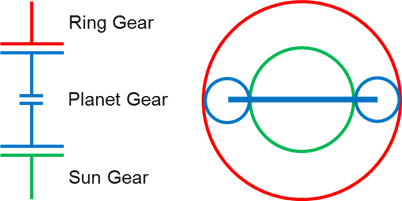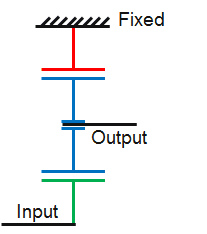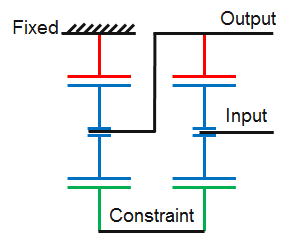# Planetary gearbox speed calculation - Simultaneous equations

• xxChrisxx
In summary, the conversation discusses the calculations and constraints involved in finding the output speed of a planetary gear train. The gear set consists of a ring gear, planet gear, and sun gear, and their rotational speeds can be calculated using a specific equation. The single planet case is relatively easy to solve, but the multiple planets case requires simultaneous solving of equations. To automate this process, using a numerical differential equation method in MATLAB is suggested.

#### xxChrisxx

I'm looking to calculate the ratio of a planetary gear train. With the ultimate goal of producing a spreadsheet/calculator to show the state of the gearbox (i.e. all shaft speeds) based on applied constraints.A gear set consists of Ring Gear, Planet Gear, Sun Gear. The sick diagram is the easiest way to show the interactions and constraints. The rotational speed of any part can be found by:

(R+S)*ωp=R*ωr + S*ωs
R - Number of teeth on ring
S - Number of teeth on sun
ωr = Ring gear speed
ωp = Planet rotational speed
ωs = Sun gear rotational speed

Single Planet CaseThis is the type of thing you'll find with a generic google. Output speed can be directly calculated based on the constraints. In this case we have three states, two constraints and one gear equation to find the output. Easy peasy.

Multiple PlanetsThis case is an example of a more tricky case. 6 unknowns, 4 constraints, 2 gear equations. This has to be solved simultaneously due to the interactions between the gear sets:
Sun Gear 1 = Sun Gear 2 = intermediate
Planet Carrier 1 = Ring Gear 2 = output.

I can do this by hand with substitution. I'd like to automate it as the cases become more complicated very quickly.

Eg 4 gear sets = 12 unknowns
4 gear equations
5 fixed constraints (due to gearbox architecture)
3 variable constraints (used to select different gears)
So there is enough information to solve it. It's just a pain doing it by hand.

What's the best way to automate this using Excel or similar?

## What is a planetary gearbox?

A planetary gearbox is a type of gear system commonly used in mechanical engineering to transmit power and alter the speed of rotation. It consists of a central gear (called the sun gear), surrounded by multiple smaller gears (called planet gears), all of which are contained within an outer ring gear (called the ring gear).

## How does a planetary gearbox work?

A planetary gearbox works by using the rotation of the sun gear to drive the rotation of the planet gears, which in turn drive the rotation of the ring gear. This creates a reduction in speed and an increase in torque, making it useful for a wide range of applications.

## What is the purpose of simultaneous equations in planetary gearbox speed calculation?

Simultaneous equations are used in planetary gearbox speed calculation to determine the relationship between the input speed, output speed, and gear ratios of the sun gear, planet gears, and ring gear. This allows for precise calculation of the output speed based on a given input speed and gear ratio.

## What factors affect the speed calculation of a planetary gearbox?

The speed calculation of a planetary gearbox is affected by several factors, including the number of teeth on each gear, the gear ratios, and the direction of rotation. The size and placement of the gears within the gearbox also play a role in the final speed calculation.

## How can I determine the optimal gear ratio for a planetary gearbox?

The optimal gear ratio for a planetary gearbox depends on the specific application and desired output speed and torque. It can be determined by using simultaneous equations to calculate the output speed for different gear ratios and selecting the one that best meets the requirements. Additionally, computer-aided design (CAD) software can be useful in simulating and testing different gear ratios before implementation.